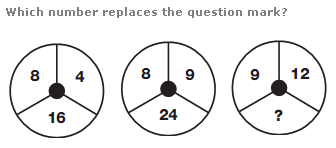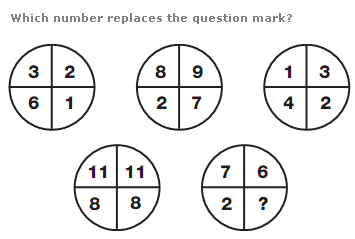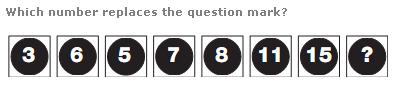# Puzzles - Number puzzles

### Exercise :: Number puzzlesAnswer : 27 Explanation : In each circle, multiply the top two numbers together, and divide by 2 for the left hand circle, 3 for the centre, and 4 for the right hand circle, putting the result in the lower segment.Answer : 5 Explanation : Take values in the top left and centre circles and put their sum in correspond- ing segments of the lower left circle. Take values in the middle and right hand circles, and put their difference into corresponding segments of the lower right circle.Answer : 22 Explanation : Working from left to right, add numbers in the first two boxes together, and subtract 4, to give the next value along.